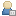10.03.2019 - Round 1 results of our "A Love Letter For FreeBASIC" game dev competition have been published. Please be sure to check the results thread: http://games.freebasic.net/forum/index.php?topic=629.0. Don't forget that the competition is continuing with a round 2, lasting till 29th of April, 300 USD first prize. Stay tuned!

###Show Posts

This section allows you to view all posts made by this member. Note that you can only see posts made in areas you currently have access to.

### Messages - Pyramidschem

Pages: 
1
##### Programming / Re: What data structure can I use to draw walls on a map?
« on: January 14, 2013, 03:33:39 PM »
Thanks a lot, I will study it and then report.

Edit1 :
Ok I'm this type of guy that needs pictures. So here what I got for begin.I didn't reach yet your nice collision function. I've to deal with the fact that I want to be able to choose the screen resolution at the execution. So the size of the map array may change.

Maybe I see one solution, it would be to design the map at his higher resolution and then fix the datas accordingly, then to make a sort of rescale function. One fact is that I need a good collision function like what you've shown above, so I've to keep the most part of your code.

I'm watching this right now. Feedback will come later.

2
##### Programming / What data structure can I use to draw walls on a map?
« on: January 13, 2013, 05:49:04 PM »
Hello again,

I am still working on my little project. I want my circles to be able to move on a labyrinth-like map ( omg i'm rewritting pacman).

As I am a very beginer, I don't know at all how to store my walls definitions. I've thought about saving only the two extremities of lines so that each time I read such a couple of coordinates in a table I will redraw the lines.

The thing that doesn't satisfy me is about collision. Doing such a table doesn't help me to save collisions tests when they are not needed. I've heard about z-buffer and so on in my very youth, but I've forgotten all of that now. Is there a simple structure I should use you are awared about, and you know perfectly working for my purpose?

Thanks if so, because it is possible to waste or gain a lot of time with those data structures depending on how you are ignorant about them.

3
##### Programming / About Min(a,b) math functions - Formula vs Compare
« on: January 13, 2013, 04:21:26 PM »
Hello,

First thanks for grant me clearance to this forum. Sorry for I am not too much fluent in english even if I borrow some game the basic vocabulary, and it is also true that google provides a correct translator...

-Edit :
I wanted to share with you a test I made in order to choose between 2 min(a,b) implementation. The first using the formula :
Code: [Select]
`    Min = ( R1 + R2 - Abs( R1 - R2) ) / 2`And the second using the case comparison
Code: [Select]
`select case R1        case Is <= R2            Min = R1        case else            Min = R2        End select `
I writed this test for that purpose :
Code: [Select]
`ScreenRes 600, 240, 16CONST AS integer Max_counter = 50000CONST AS integer Max_number = 1CONST AS integer Max_DurationArray = 500Declare Sub Test_Min_formula()Declare Sub Test_Min_CaseCompare()Declare Function Sum_Mean( Arr() as integer) as doubleDim shared AS double Timer_Start , Timer_End, DurationDim shared AS Integer _    DurationCaseCompare(Max_DurationArray),_    DurationFormula(Max_DurationArray)Dim shared AS integer aaa_counter, bbb_counter'***For bbb_counter = 0 to Max_DurationArray        Randomize Timer , 1        '---Test for Case compare method        Timer_Start = Timer        For aaa_counter = 1 to Max_counter            Test_Min_CaseCompare()            Next aaa_counter        Timer_End = Timer        Duration = Timer_End - Timer_Start                DurationCaseCompare(bbb_counter)=Duration*100000        Locate 1,1 : ? "Duration for case compare = ";_        DurationCaseCompare(bbb_counter)        '---Test for Direct Formula method        Timer_Start = Timer        For aaa_counter = 1 to Max_counter            Test_Min_formula()            Next aaa_counter        Timer_End = Timer        Duration = Timer_End - Timer_Start                DurationFormula(bbb_counter)=Duration*100000        Locate 5,1 : ? "Duration for formula = ";_        DurationFormula(bbb_counter)Next bbb_counter'RESULTSLocate 10,1 : ? "CaseCompare_mean = ";_Sum_Mean(DurationCaseCompare())Locate 11,1 : ? "Formula_mean = ";_Sum_Mean(DurationFormula())Locate 18,1 : ? "Factor diff. = ";_Sum_Mean(DurationFormula())/Sum_Mean(DurationCaseCompare())While Inkey <> "": Wend Sleep_ . _ . _ . _ . _ . _ . _ . _ . _ . Sub Test_Min_formula()Dim R1 As doubleDim R2 As doubleDim Min As double    R1 = 100 * Rnd    R2 = 100 * Rnd    Min = ( R1 + R2 - Abs( R1 - R2) ) / 2    End SubSub Test_Min_CaseCompare()Dim R1 As doubleDim R2 As doubleDim Min As double      R1 = 100 * Rnd    R2 = 100 * Rnd    select case R1        case Is <= R2            Min = R1        case else            Min = R2        End select    End SubFunction Sum_Mean(Arr() as integer) as doubleDim AS double sum = 0For bbb_counter = 0 to Max_DurationArray    sum = Arr(bbb_counter) + sumNext bbb_counterSum_Mean = sum / (Max_DurationArray+1)End function`
RESULT :

I found that the comparison using SELECT CASE was slightly faster. I have the duration of the min computation take 1.03 more time than the SelectCase usage.

Do you agree with that?

Pages: